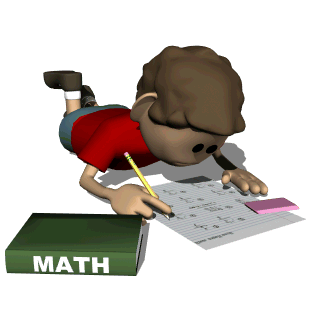# Math ~ Unit 3

• Unit 3: OperationsIn this unit, our class will:

• Find inputs, outputs, and rules in "What's My Rule?" tables;
• Use "close but easier" numbers and mental math;
• Estimate and use partial-sums addition to solve problems;
• Estimate and use column addition to solve problems;
• Use multiples of 100s, 10s, and 1s to count up;
• Use expand and trade subtraction;
• Complete a picture graph with symbols representing the data;
• Create arrays and determine products for multiplication squares;
• Generate pairs of products that demonstrate the turn-around rule;
• Use adding-a-group strategy to solve multiplication facts;
• Use subtracting-a-group strategy to solve multiplication facts;
• Write equivalent names using addition, subtraction, and multiplication;
• Multiply and divide within 100 fluently;
• Know all products of 1 digit numbers multiplied by 1, 2, 5, and 10;
• Add and subtract fluently within 1, 000

Everyday Mathematics Games:

Throughout Unit 3, our class will play the games listed below to practice skills and developing strategic thinking.  Check your child's homework folder for information as we play these games in class!

• Roll to 1, 000 (see Student Reference Book page 253)
• Shuffle to 100 (see Student Reference Book page 256)
• Shuffle to 1, 000 (see Student Reference Book page 256-257)
• Array Bingo (see Student Reference Book page 232)
• Multiplication Draw (see Student Reference Book page 248)
• Name that Number (see Student Reference Book page 249)

### Choose one of the sites below to find great activities to work on:

• Math Baseball- Practice your addition/subtraction skills by playing a game of baseball.

• Math 500 Race- Practice Addition/Subtraction and Multiplication/Division as you race your car around the track!  What is your fastest score??  (Press "Start Your Engine" to begin)

• Math Fact Cafe- Practice multiplication and division facts using flashcards (scroll to the middle of the page to find the "flashcards"